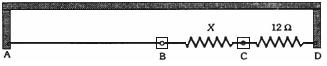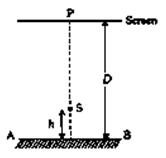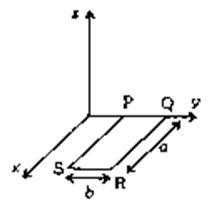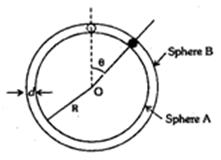Click to Chat

1800-1023-196

+91-120-4616500

CART 0

• 0

MY CART (5)

Use Coupon: CART20 and get 20% off on all online Study Material

ITEM
DETAILS
MRP
DISCOUNT
FINAL PRICE
Total Price: Rs.

There are no items in this cart.
Continue Shopping• Complete JEE Main/Advanced Course and Test Series
• OFFERED PRICE: Rs. 15,900
• View Details

```IIT-JEE-Physics-Mains-2002

MAIN
Time : Two hours                                                                             Max. Marks : 60
______________________________________________________________

Instructions
1.     The Question Paper has 4 printed pages and has 12 questions. Please ensure that the copy of the Question Paper you have received contains all the questions.
2.     Attempts ALL questions. Each question carries 5 marks.
3.     Answer each question starting on a new page. The corresponding Question number must be written in the left margin.
4.     Do not write more than one answers for the same question. In case you attempt a question more than once, please cancel the answer(s) you consider to be wrong. Otherwise, only the answer appearing last will be evaluated.
5.     Use only Arabic numerals (0, 1, 2 .............9) in answering the questions irrespective of the language in which your answer.
6.     Use of logarithmic tables is NOT PERMITTED.
7.     Use of calculators is NOT PERMITTED.
8.     Both magnitude and direction of vector quantities, if any, in your answer should be indicated clearly.
9.     Irrational numbers, if any, in your answer need not be expressed in decimal form.
10.  If the final answer appears in the form of a ratio or a product of two numbers, that need not to be further simplified.
_______________________________________________________________

1.         Two narrow cylindrical pipes A and B have the same length. Pipe A is open at both ends and is filled with a monoatomic gas of molar mass MA. Pipe B is open at one end and closed at the other end, and is filled with a diatomic gas of molar mass MB. Both gases are at the same temperature.
(a)       If the frequency to the second harmonic of the fundamental mode in ppe A is equal of the frequency of the third harmonic of the fundamental mode in pipe B, determine the value of MA/MB.
(b)       Now the open end of pipe B is also closed (so that the pipe is closed at both ends. Find the ratio of the fundamental frequency in pipe A to that in pipe B.

2.         A cubical box of side 1 meter contains helium gas (atomic weight 4) at a pressure of 100 N/m2. During an observation time of 1 second, an atom travelling with root-mean-square speed parallel to one of the edges of the cube, was found to make 500 hits with a particular wall, without any collision with other atoms. Take R = 25/3 J/mol-K and k = 1.38 × 10-23 J/K.
(a)       Evaluate the temperature of the gas.
(b)       Evaluate the average kinetic energy per atom.
(c)        Evaluate the total mass of helium gas in the box.

3.  A uniform solid cylinder of density 0.8 g/cm3 floats in equilibrium in a combination of two non-mixing liquids A and B with its axis vertical. The densities of the liquids A and B are 0.7 g/cm3 and 1.2 g/cm2, respectively. The height of liquid A is hA = 1.2 cm. The length of the part of the cylinder immersed in liquid B is
hB = 0.8 cm.(a)       Find the total force exerted by liquid A on the cylinder.
(b)       Find h, the length of the part of the cylinder in air.
(c)        The cylinder is depressed in such a way that its top surface is just below the upper surface of liquid A and is then released. Find the acceleration of the cylinder immediately after it is released.

4.    A thin uniform wire AB of length 1 m, an unknown resistance X and a resistance of 12 W are connected by thick conducting strips, as shown in the figure. A battery and a galvanometer (with a sliding jockey connected to it) are also available. Connections are to be made to measure the unknown resistance X using the principle of Wheatstone bridge. Answer the following questions.(a)       Are there positive and negative terminals on the galvanometer?
(b)       Copy the figure in your answer book and show the battery and the galvanometer (with jockey) connected at appropriate points.
(c)        After appropriate connections are made, it is found that no deflection takes place in the galvanometer when the sliding jockey touches the wire at a distance of 60 cm from A. Obtain the value of the resistance X.

5.         A hydrogen-like atom (described by the Bohr model) is observed to emit six wavelengths, originating from all possible transitions between a group of levels. These levels have energies between -0.85 eV and -0.544 eV (including both these values).
(a)       Find the atomic number of the atom.
(b)       Calculate the smallest wavelength emitted in these transitions.
(Take hc = 1240 eV-nm, ground state energy of hydrogen atom = -13.6 eV.

6.         A point source S emitting light of wavelength 600 nm is placed at a very small height h above a flat reflecting surface AB (see figure). The intensity of the reflected light is 36% of the incident intensity. Interference fringes are observed on a screen placed parallel to the reflecting surface at a very large distance D from it.(a)       What is the shape of the interference fringes on the screen?
(b)       Calculate the ratio of the minimum to the maximum intensities in the interference fringes formed near the point P (shown in the figure).
(c)        If the intensity at point P corresponds to a maximum, calculate the minimum distance through which the reflecting surface AB should be shifted so that the intensity at P again becomes maximum.

7.         A rectangular loop PQRS made from a uniform wire has length a, width b and mass m. It is free to rotate about the arm PQ, which remains hinged along a horizontal line taken as the y-axis (see figure). Take the vertically upward direction as the z-axis. A uniform magnetic field vector B = (3i + 4k) B0 exists in the region. The loop is held in the ex-y plane and a current I is passed through it. The loop is now released and is found to stay in the horizontal position in equilibrium.(a)       What is the direction of the current I in PQ?
(b)       Find the magnetic force on the arm RS.
(c)        Find the expression for I in terms of B0, a, b and m

8.         Two metallic plates A and B, each of are 5 × 10-4 m2, are placed parallel to each other at a separation of 1 cm. Plate B carries a positive charge of 33.7 × 10-12C. A monochromatic beam of light, with photons of energy 5 eV each, starts falling on plate A at t = 0 so that 106 photons fall on it per square meter per second. Assume that one photoelectron is emitted for every 106 incident photons. Also assume that all the emitted photoelectrons are collected by plate B and the work function of plate A remains constant at the value 2 eV. Determine
(a)       the number of photoelectrons emitted up to t = 10s.
(b)       the magnitude of the electric field between the plates A and B at t = 10 s and
(c)        The kinetic energy of the most energetic photoelectron emitted at t = 10 s when it reaches plate B.
Neglect the time taken by the photoelectron to reach plate B.
Take e0 = 8.85 × 10-12 C2/N - m2.

9.         A metal bar AB can slide on two parallel thick metallic rails separated by a distance l. A resistance R and an inductance L are connected to the rails as shown in the figure. A long straight wire carrying a constant current I0 is placed in the plane of the rails and perpendicular to them as shown. The bar AB is held at rest at a distance x0 from the long wire. At t = 0, it is made to slide on the rails away from the wire. Answer the following questions.(a)       Find a relation among i, di/dt and dΦ/dt , where I is the current in the circuit and F is the flux of the magnetic field due to the long wire through the circuit.
(b)       It is observed that at time t = T, the metal bar AB is at a distance of 2x0from the long wire and the resistance R carries a current i1. Obtain an expression for the net charge that has flown through resistance R from
t = 0 to t = T.
(c)        The bar is suddenly stopped at time T. The current through resistance R is found to be i1/4 at time 2T. Find the value of L/R in terms of the other given quantities.

10.       On a frictionless horizontal surface, assumed to be the x-y plane, a small trolley A is moving along a straight line parallel to the y-axis (see figure) with a constant velocity of (√3 - 1) m/s. At a particular instant, when the line OA makes an angle of 45o with the x-axis, a ball is thrown along the surface from the origin O. Its velocity makes an angle f with the x-axis and it hits the trolley.(a)       The motion of the ball is observed from the frame of the trolley. Calculate the angle q made by the velocity vector of the ball with the x-axis in this frame.
(b)       Find the speed of the ball with respect to the surface, if f = 4q/3.

11.       A spherical ball of mass m is kept at the highest point in the space between two fixed, concentric spheres A and B (see figure). The smaller sphere A has a radius R and the space between the two spheres has a width d. The ball has a diameter very slightly less then d. All surfaces are frictionless. The ball is given a gentle push (towards the right in the figure). The angle made by the radius vector of the ball with upward vertical is denoted by q (shown in the figure).(a)       Express the total normal reaction force exerted by the spheres on the ball as a function of angle q.
(b)       Let NA and NB denote the magnitudes of the normal reaction forces on the ball exerted by the spheres A and B, respectively. Sketch the variation of NA and NBas functions of cos q in the range 0 < q < p by drawing two separate graphs in your answer book, taking cos q on the horizontal axes.

12.       Three particles A, B and C, each of mass m, are connected to each other by three massless rigid rods to form a rigid, equilateral triangular body of side l. This body is placed on a horizontal frictionless table (x - y plane) and is hinged to it at the point A so that it can move without friction about the vertical axis through A (see figure). The body is set into rotational motion on the table about A with a  constant angular velocity w.(a)       Find the magnitude of the horizontal force exerted by the hinge on the body.
(b)       At time T, when the side BC is parallel to the x-axis, a force F is applied on B along BC (as shown). Obtain the x-component and the y-component of the force exerted by the hinge on the body, immediately after time T.
```### Course Features

• 731 Video Lectures
• Revision Notes
• Previous Year Papers
• Mind Map
• Study Planner
• NCERT Solutions
• Discussion Forum
• Test paper with Video Solution